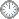“执行定理”的证明(d+)

This is an in-mail from TYUST.
新入の者--> What is going on ? (redirected)new

(接前: 10 04 03) “执行定理” 的证明(d+).
.
There is a prime divisor T on birational models of X such that a(T, X, B + sL) = eps'.
.
18 19 24 25
|    |   |   |
para 4.1
|
28
.

---- 18, 19, 24, 25: X, B, L, s. (已定义)
---- 28: T.
.
Let x be the generic point of the centre of T on X.
.
18  28
|     |
para 4.2
|
29
.

---- 29:  x 为 T 的中心的母点.
.
Assume x is not a closed point.
.
18
|
para 4.3
|
30
.

---- 30: x 非闭.
.
Then cutting by general elements of |A| and applying induction, there is a positive number v bounded from below away from zero such that (X, B + vL) is lc near x.
.
0     i
|     |
para 4.4
|     |
31  32
.

---- 0: 归纳假设.
---- i:  cutting by general elements of |A|.
(红色表示特定技术)
---- 31: v 有正下界.
---- 32: (X, B + vL) lc near x.
.
Then (X, B + (1 - eps'/eps)vL) is eps'-lc near x, by Lemma 2.3, because B + (1 - eps'/eps)vL = eps'/eps B + (1 - eps'/eps) (B + vL).
.
0  32
|   |   |
para 4.5
|
33
.

---- 33(X, B + (1 - eps'/eps)vL) eps'-lc near x.
.
In particular, s  (1 - eps'/eps)v.
.
25  33
|     |
para 4.6
|
34
.

---- 34: (1 - eps'/eps)v.
.
Thus we can assume x is a closed point.
.
?
|
para 4.7
|
35
.

---- 35: x 系 closed point.
.

.

符号大全上下标.|| 常用：↑↓ π ΓΔΛΘΩμφΣ∈  ∪ ∩ ⊆ ⊇ ⊂ ⊃ ≤ ≥ ⌊ ⌋ ⌈ ⌉ ≠ ≡ ⁻⁰ ¹ ² ³ ᵈ ₀ ₁ ₂ ₃ ᵢ .

Glossary(AG)

*

Introduction
....
....
11/9
...
Proposition 5.5 11/5

http://blog.sciencenet.cn/blog-315774-1198012.html

全部精选博文导读

GMT+8, 2019-10-21 08:45